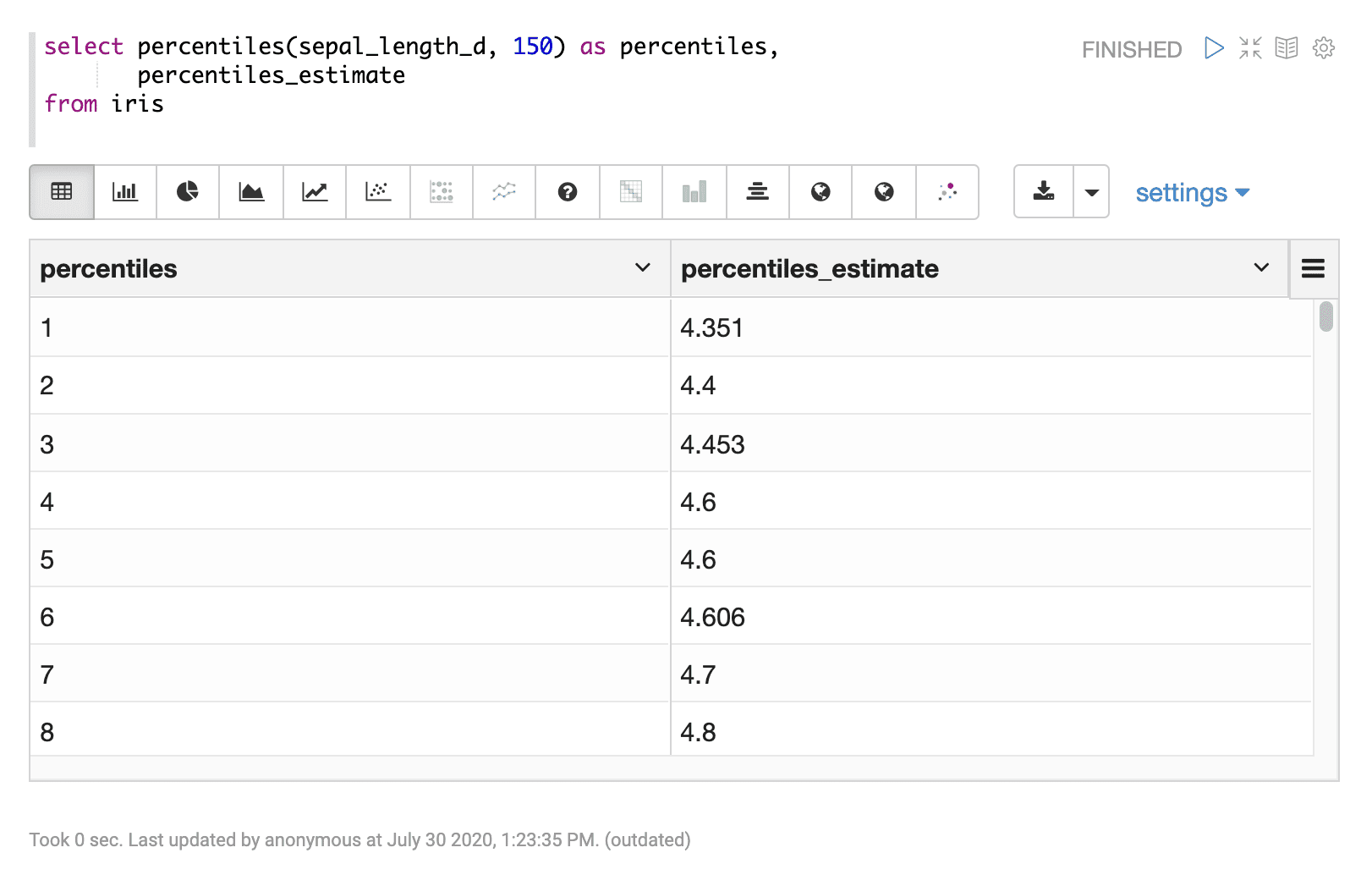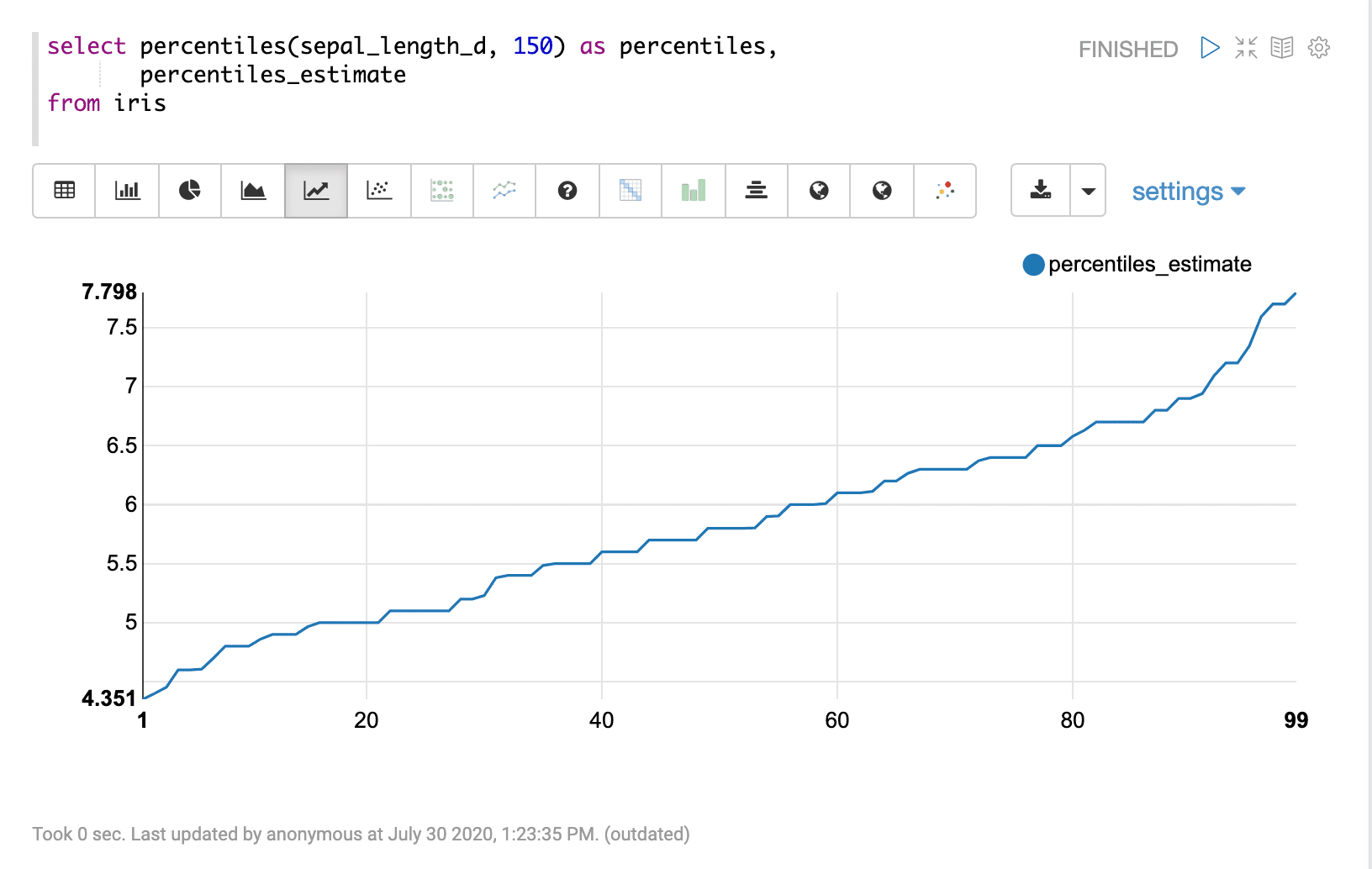# Percentiles (percentiles)

The `percentiles` function can be used to visualize the estimated percentiles (1-99) for a numeric field. The `percentiles` function takes two parameters:

1. The numeric field to compute the percentiles for

2. The sample size

## Sample Syntax

``````select percentiles(sepal_length_d, 150) as percentiles,
percentiles_estimate
from iris``````

## Result set

The result set for the `percentiles` function contains one row for each percentile 1-99. The `percentiles` function returns the percentile (1-99). The `percentiles_estimate` field contains the estimated percentile value at each percentile.

The percentiles are calculated for a random sample that matches the `WHERE` clause. If the is no `WHERE` clause the samples are taken from the entire data set.

Sample result set in Apache Zeppelin## Visualization

The percentiles can be visualized by plotting the percentile (1-99) on the x-axis and the percentile estimate on the y-axis.

Sample visualization of percentiles in Apache Zeppelin: